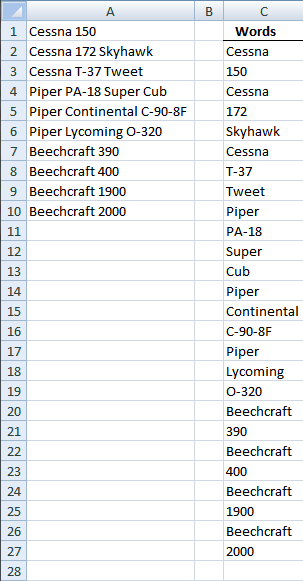Author: Oscar Cronquist Article last updated on December 30, 2018

This post describes how to split words in a cell range into a cell each using a custom function. I hope this picture explains it all:The cell range is A1:A10 and contain words.

### The array formula in C2:C27 contains an udf:

1. Select all the cells to be filled, C2:C27
2. Type the array formula SplitWords(\$A\$1:\$A\$10) into the formula bar.

### How to create an array formula

1. Copy (Ctrl + c) and paste (Ctrl + v) array formula into formula bar.
2. Press and hold Ctrl + Shift.
3. Press Enter once.
4. Release all keys.

User defined function: Read Rick Rothstein's (MVP - Excel) comment!

```
Function SplitWords(rng As Range) As Variant()

Dim x As Variant, Wrds() As Variant, Cells_row As Long
Dim Cells_col As Long, Words As Long, y() As Variant
ReDim y(0)
Wrds = rng.Value
For Cells_row = LBound(Wrds, 1) To UBound(Wrds, 1)
For Cells_col = LBound(Wrds, 2) To UBound(Wrds, 2)
x = Split(Wrds(Cells_row, Cells_col))
For Words = LBound(x) To UBound(x)
y(UBound(y)) = x(Words)
ReDim Preserve y(UBound(y) + 1)
Next Words
Next Cells_col
Next Cells_row

SplitWords = Application.Transpose(y)

End Function

```

### Where to copy the code

1. Press with left mouse button on "Developer" tab on the ribbon
How to enable developer tab on the ribbon
2. Press with left mouse button on "Visual Basic" button
3. Insert a new module
4. Copy this udf example and paste it into new module

### Get the Excel fileextract-words-from-a-range-udf.xls